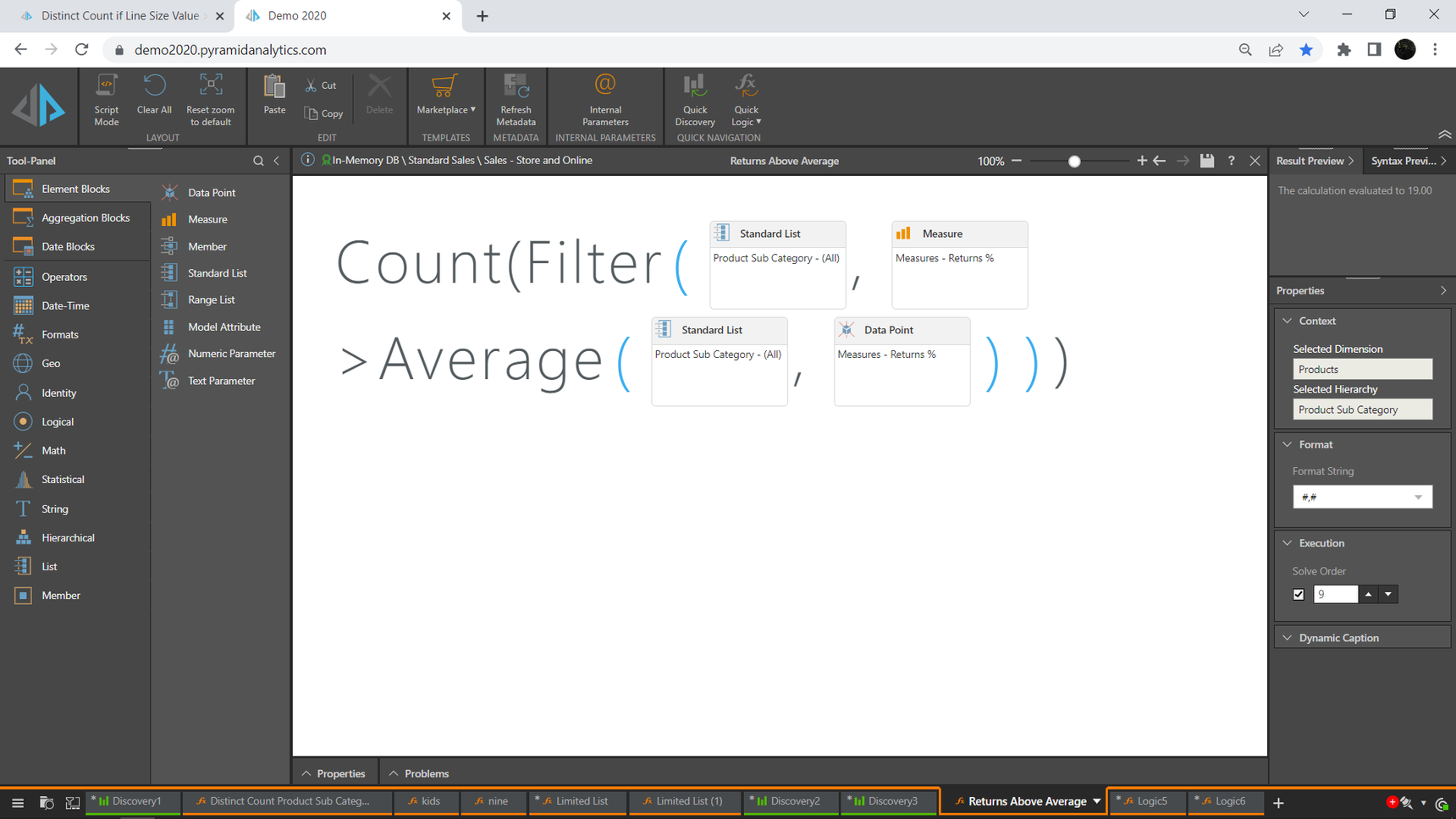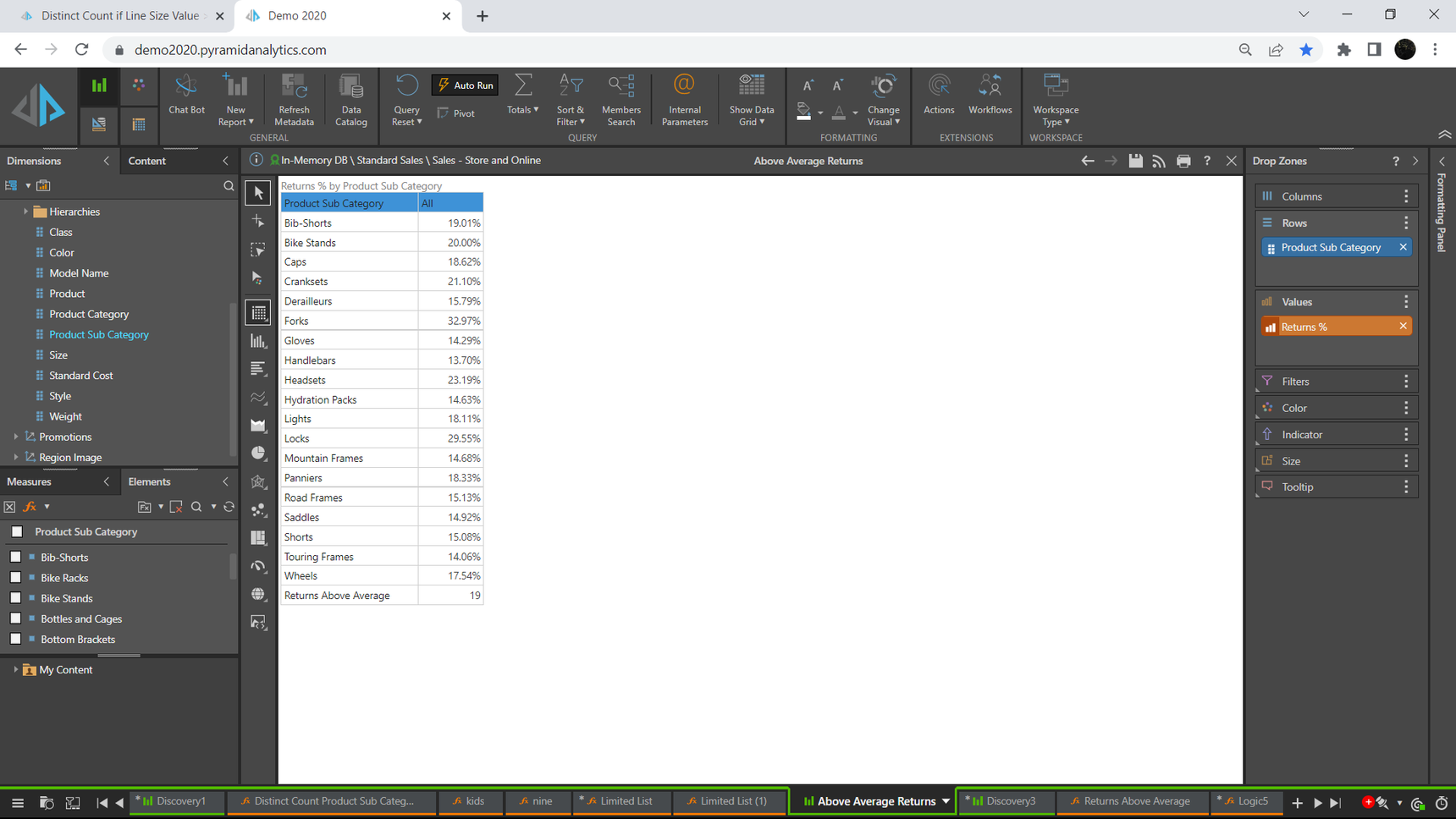0# Distinct Count if Line Size Value >= Max Line Size for Group

Hi

So I have a measure showing count(distinct aggregate reference) and it works as expected but now I need to only count if the line size value is greater than the maximum size allowed for the subgroup of that risk. I know how to do it with specific filter i.e. line sizes values > X using parameter but is it possible to do it based on another measure?

Thanks

nick

2replies Oldest first
• Oldest first
• Popular•• Team Lead - Product Management
• Ian_Macdonald
• 1 mth ago
• Reported - view

Hi Nick,

Yes, is the simple answer. How exactly you do it depends on your model structure. Can you share a little more of that and how you calculate the permitted line size value? If you have that, then you can use it in your filter or other comparison function.

You may, however, have to stray into Script Mode to achieve this as the Set / Calculation graphical editor may not cater for this level of detail.

Here's an example where I am filtering my list of Product Sub Categories to those whose Returns % are above the average Returns %. Returns % is itself a calculated Measure of Returns / Units Sold (I've substituted the Measure name Returns % instead of the GUID for clarity):

``{Filter({AllMembers([Products].[Product Sub Category])}, [measures].[Returns%] > Average(AllMembers([Products].[Product Sub Category]), [measures].[Returns %]))}``

We can then count this list thus:

``Count({Filter({AllMembers([Products].[Product Sub Category])}, [measures].[Returns%] > Average(AllMembers([Products].[Product Sub Category]), [measures].[Returns %]))})``

We can do the Count graphically:Note the Execution Solve Order set to a value to make sure the Count is calculated correctly and the correct formatting applied  in the Discover below:Hope that helps.

Ian

Like 1
Like Follow